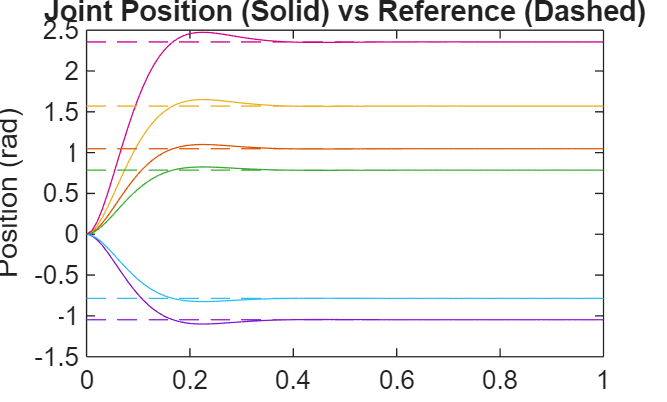jointSpaceMotionModel

Model rigid body tree motion given joint-space inputs

Description

The jointSpaceMotionModel object models the closed-loop joint-space motion of a manipulator robot, specified as a rigidBodyTree object. The motion model behavior is defined by the MotionType property.

For more details about the equations of motion, see Joint-Space Motion Model.

Creation

Description

motionModel = jointSpaceMotionModel creates a motion model for a default two-joint manipulator.

example

motionModel = jointSpaceMotionModel("RigidBodyTree",tree) creates a motion model for the specified rigidBodyTree object.

motionModel = jointSpaceMotionModel(Name,Value) sets additional properties specified as name-value pairs. You can specify multiple properties in any order.

Properties

expand all

Rigid body tree robot model, specified as a rigidBodyTree object that defines the inertial and kinematic properties of the manipulator.

Natural frequency of error dynamics, specified as a scalar or n-element vector in Hz, where n is the number of nonfixed joints in the associated rigidBodyTree object in the RigidBodyTree property.

Dependencies

To use this property, set the MotionType property to "ComputedTorqueControl" or "IndependentJointMotion".

Damping ratio of the second-order error dynamics, specified as a scalar or n-element vector of real values, where n is the number of nonfixed joints in the associated rigidBodyTree object in the RigidBodyTree property. If a scalar is specified, then DampingRatio becomes an n-element vector of value s, where s is the specified scalar.

Dependencies

To use this property, set the MotionType property to "ComputedTorqueControl" or "IndependentJointMotion".

Proportional gain for proportional-derivative (PD) control, specified as a scalar or n-by-n matrix, where n is the number of nonfixed joints in the associated rigidBodyTree object in the RigidBodyTree property. You must set the MotionType property to "PDControl". If a scalar is specified, then Kp becomes s*eye(n), where s is the specified scalar.

Dependencies

To use this property, set the MotionType property to "PDControl".

Derivative gain for PD control, specified as a scalar or n-by-n matrix, where n in the number of nonfixed joints in the rigidBodyTree object in the RigidBodyTree property. If a scalar is specified, then Kp becomes s*eye(n), where s is the specified scalar.

Dependencies

To use this property, set the MotionType property to "PDControl".

Type of motion, specified as a string scalar or character vector that defines the closed-loop joint-space behavior that the object models. Options are:

• "ComputedTorqueControl" — Compensates for full-body dynamics and assigns the error dynamics specified in the NaturalFrequency and DampingRatio properties.

• "IndependentJointMotion" — Models each joint as an independent second-order system using the error dynamics specified by the NaturalFrequency and DampingRatio properties.

• "PDControl" — Uses proportional-derivative control on the joints based on the specified Kp and Kd properties.

Object Functions

 derivative Time derivative of manipulator model states updateErrorDynamicsFromStep Update values of NaturalFrequency and DampingRatio properties given desired step response

Examples

collapse all

This example shows how to create and use a jointSpaceMotionModel object for a manipulator robot in joint-space.

Create the Robot

Set Up the Simulation

Set the timespan to be 1 s with a timestep size of 0.01 s. Set the initial state to be the robots, home configuration with a velocity of zero.

tspan = 0:0.01:1;
initialState = [homeConfiguration(robot); zeros(7,1)];

Define the a reference state with a target position, zero velocity, and zero acceleration.

targetState = [pi/4; pi/3; pi/2; -pi/3; pi/4; -pi/4; 3*pi/4; zeros(7,1); zeros(7,1)];

Create the Motion Model

Model the system with computed torque control and error dynamics defined by a moderately fast step response with 5% overshoot.

motionModel = jointSpaceMotionModel("RigidBodyTree",robot);
updateErrorDynamicsFromStep(motionModel,.3,.05);

Simulate the Robot

Use the derivative function of the model as the input to the ode45 solver to simulate the behavior over 1 second.

[t,robotState] = ode45(@(t,state)derivative(motionModel,state,targetState),tspan,initialState);

Plot the Response

Plot the positions of all the joints actuating to their target state. Joints with a higher displacement between the starting position and the target position actuate to the target at a faster rate than those with a lower displacement. This leads to an overshoot, but all of the joints have the same settling time.

figure
plot(t,robotState(:,1:motionModel.NumJoints));
hold all;
plot(t,targetState(1:motionModel.NumJoints)*ones(1,length(t)),"--");
title("Joint Position (Solid) vs Reference (Dashed)");
xlabel("Time (s)")